Definitions of Square Dance Calls and Concepts
Shake & Rattle [C3B]Index -->  Plus  |  A1  |  A2  |  C1  |  C2  |  C3A  |  C3B  |  C4  |  NOL  |
Definitions (Text Only) -->  Plus  |  A1  |  A2  |  C1  |  C2  |  C3A  |  C3B  |  C4  |  NOL  |
 Find call:

 \$B8@8l(B2 x 2 \$B\$+\$i(B.

Leaders \$B\$O(B Shakedown \$B\$N<+J,\$N%Q!<%H\$r9T\$\$(B,Trailers \$B\$O(B Reverse Split Swap \$B\$N<+J,\$N%Q!<%H\$r9T\$\$\$^\$9(B.

2 x 2 \$B\$G=*\$o\$j\$^\$9(B.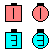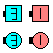Shake & Rattle\$B\$NA0(B \$B8e(B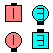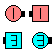Shake & Rattle\$B\$NA0(B \$B8e(B

\$BCm

• Shake & Rattle \$B\$G\$O(B, \$BA40w(B 2 x 2 \$B\$NCf\$G(B, \$B;~7W2s\$j\$K(B 1 position \$BF0\$-(B, 2 x 2 \$B\$N(B center \$B\$KBP\$7\$F\$N
• \$BA40w(B Roll \$B\$G\$-\$^\$9(B.
• Shakedown [C1] (Lee Kopman 1976): \$BGXCf9g\$o\$;\$N%+%C%W%k\$^\$?\$OE,@Z\$J(B T-Bone \$B\$N(B 2 x 2 (\$BA40w\$,(B Leads) \$B\$+\$i!%(B 1 \$B\$D\$NF0\$-\$H\$7\$F(B, Belles \$B\$O(B 1/4 Right & Run \$B\$r(B, Beaus Run & 1/4 Right (Roll) \$B\$r\$7\$^\$9!%(B \$B8~\$+\$\$9g\$C\$?%+%C%W%k\$G=*\$o\$j\$^\$9!%(B

Reverse Split Swap [C2] ("Skinny" Hall 1968): \$B8~\$+\$\$9g\$C\$?%+%C%W%k\$+\$i(B. 1 \$B\$D\$NF0\$-\$H\$7\$F(B, Beaus \$B\$O(B Circulate & 1/4 Left \$B\$r(B, Belles \$B\$O(B 1/4 Left & Circulate \$B\$r\$7\$^\$9(B. \$BGXCf9g\$o\$;\$N%+%C%W%k\$G=*\$o\$j\$^\$9(B.

Single Shake & Rattle [C3BV]: Tandem \$B\$N?M\$+\$i(B, \$B8~\$+\$\$9g\$C\$??M\$+\$i(B, \$B\$^\$?\$OGXCf9g\$o\$;\$N?M\$+\$i(B. Leaders \$B\$O(B Single Shakedown (Right Roll To A Wave and Roll) \$B\$r(B, Trailers \$B\$O(B Single Reverse Split Swap (Touch and 1/4 Out) \$B\$r\$7\$^\$9(B.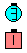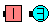Single Shake & Rattle\$B\$NA0(B \$B8e(BChoreography for Shake & RattleComments? Questions? Suggestions?